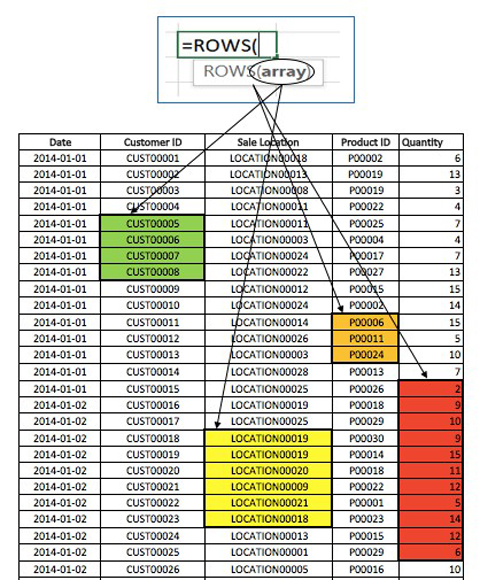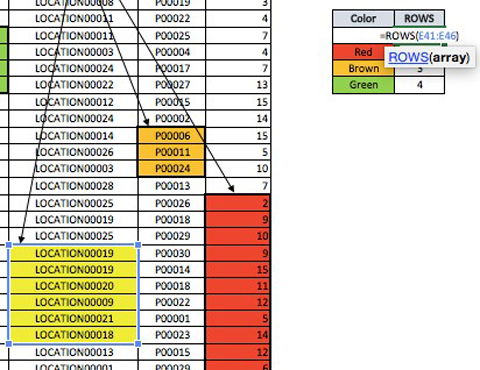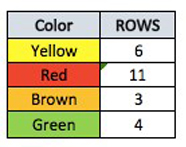ROWS FORMULA in Excel
Tuesday, Jul 14# How to Use the ROWS FORMULA in Excel

The ROWS-formula is used to count the number of rows that are selected in a range. The formula is very simple, just select the array or the table which contains the rows that you want to count within the brackets of the row formula.

Formula explanation:

Array: The cells which are selected to find out the number of rows within the selected area.

Example:

In this example, we are going to find out the number of rows from the selected cells from the following data table.Formula Implementation:Result:Ultimately, if we want to count the number of rows associated with some specific data, we can do so using the rows formula.

I hope that helps. Please leave a comment below with any questions or suggestions. For more in-depth Excel training, checkout our Ultimate Excel Training Course here. Thank you!

Article RatingInline FeedbacksLearn 10 great Excel techniques that will wow your boss and make your co-workers say, “how did you do that??”